# ICSE Class 8 Maths Selina Solutions Chapter 6 Sets

Chapter 6 of ICSE Class 8 Maths is all about the “Sets”. For the first time, one German mathematician Georg Cantor encountered sets while working on trigonometric problems. After that, he developed the theory of sets. In this chapter, students will learn to find the union and intersection of sets, definition of disjoint sets and finding the complement of a set.

Students must solve all the questions provided in the exercise to understand the applications of sets in a better way. Also, it will give confidence to the students to solve any types of problems related to sets during the annual exams. Students can download the ICSE Class 8 Maths Selina Solutions Chapter 6 Sets from the link provided below. In case students get stuck in solving the exercise problems then they can refer to the solution pdf. The Selina Solutions are prepared by subject experts and are explained in steps so that students can easily get the concept.

## Download ICSE Class 8 Maths Selina Solutions PDF for Chapter 6:-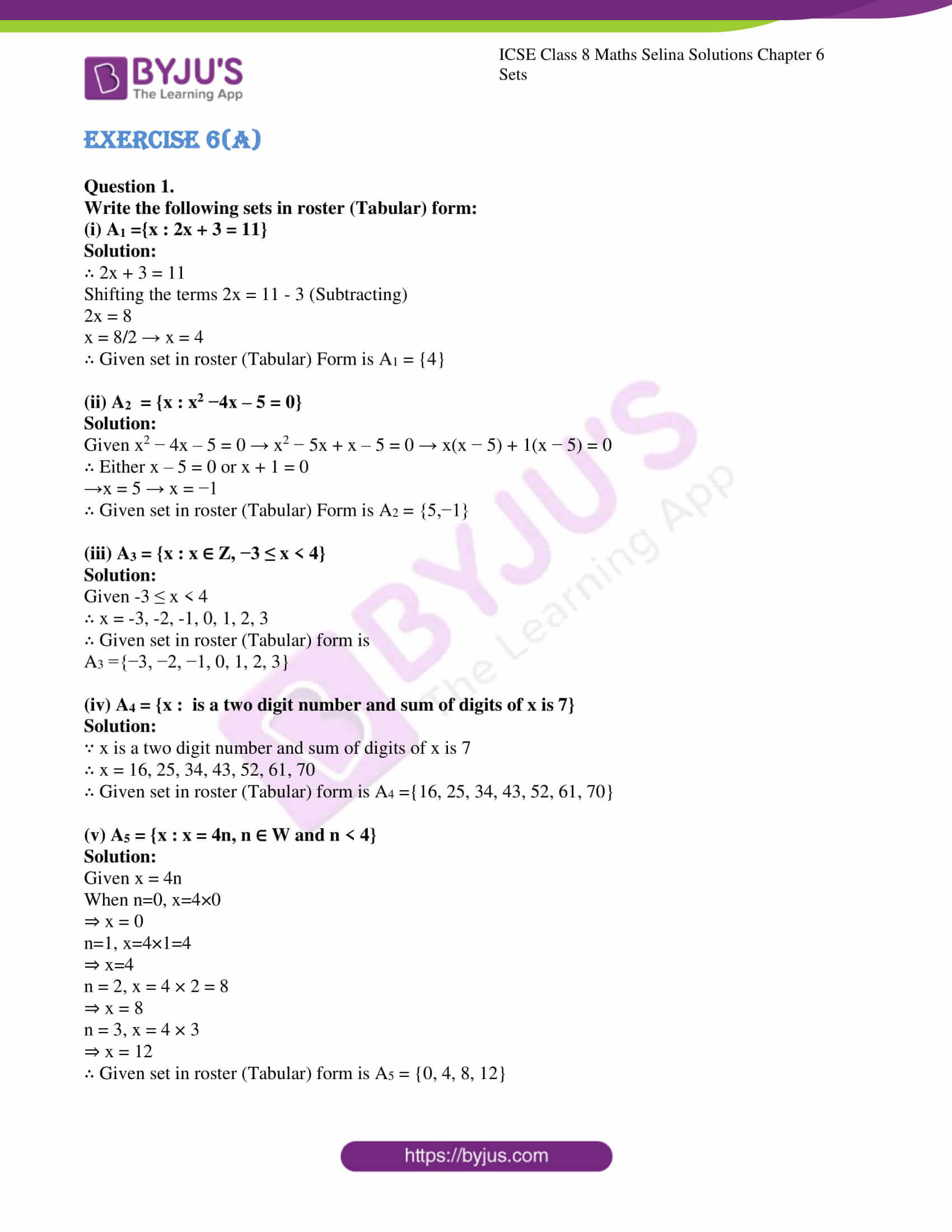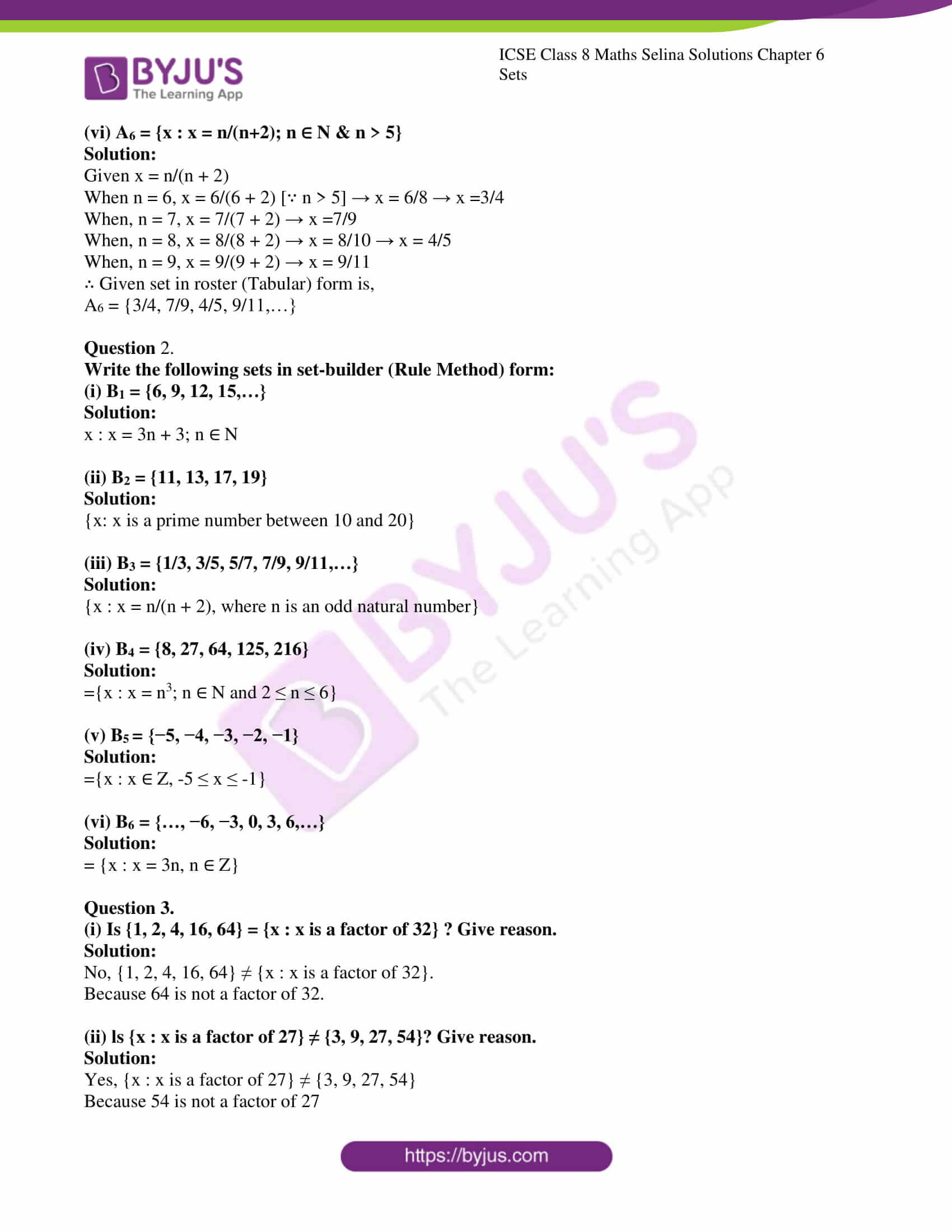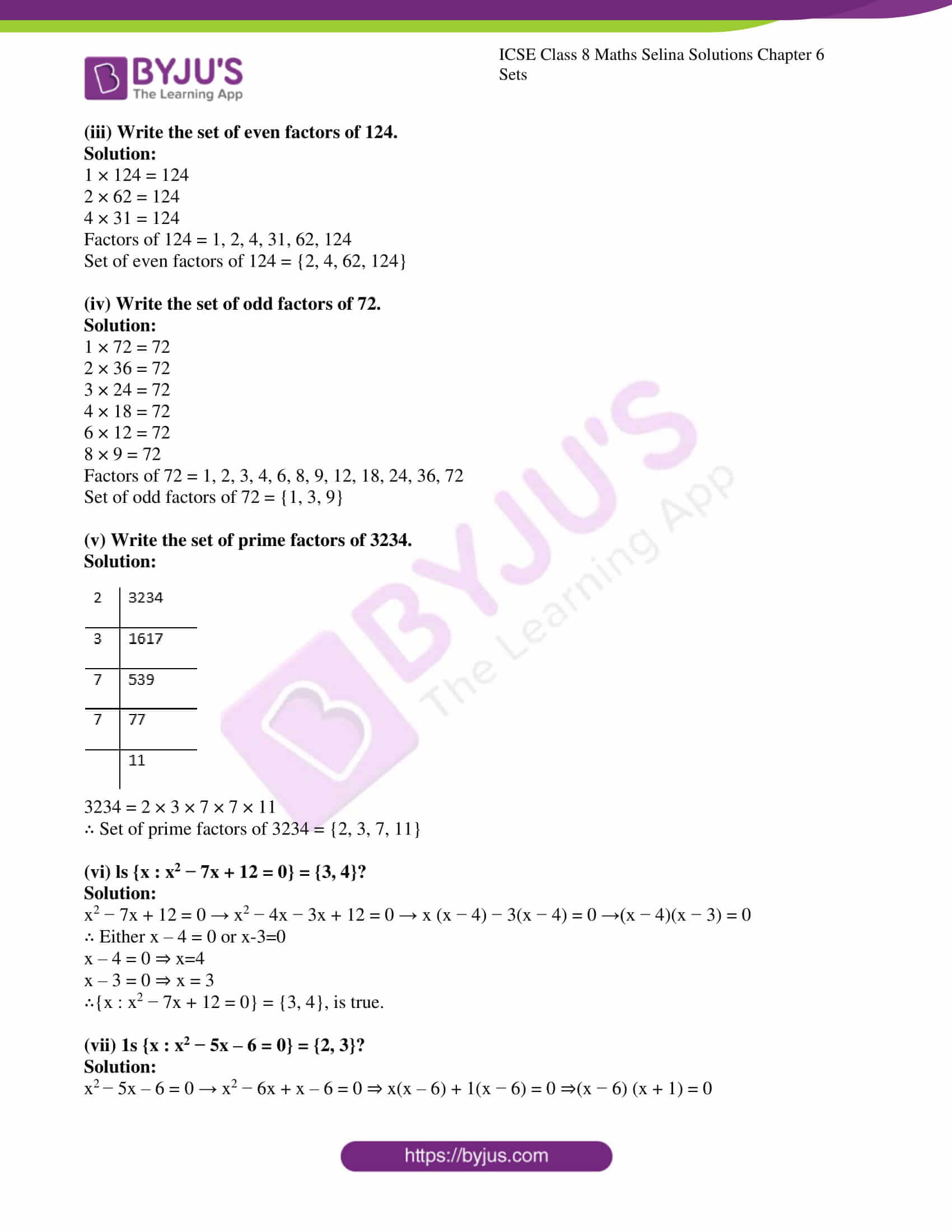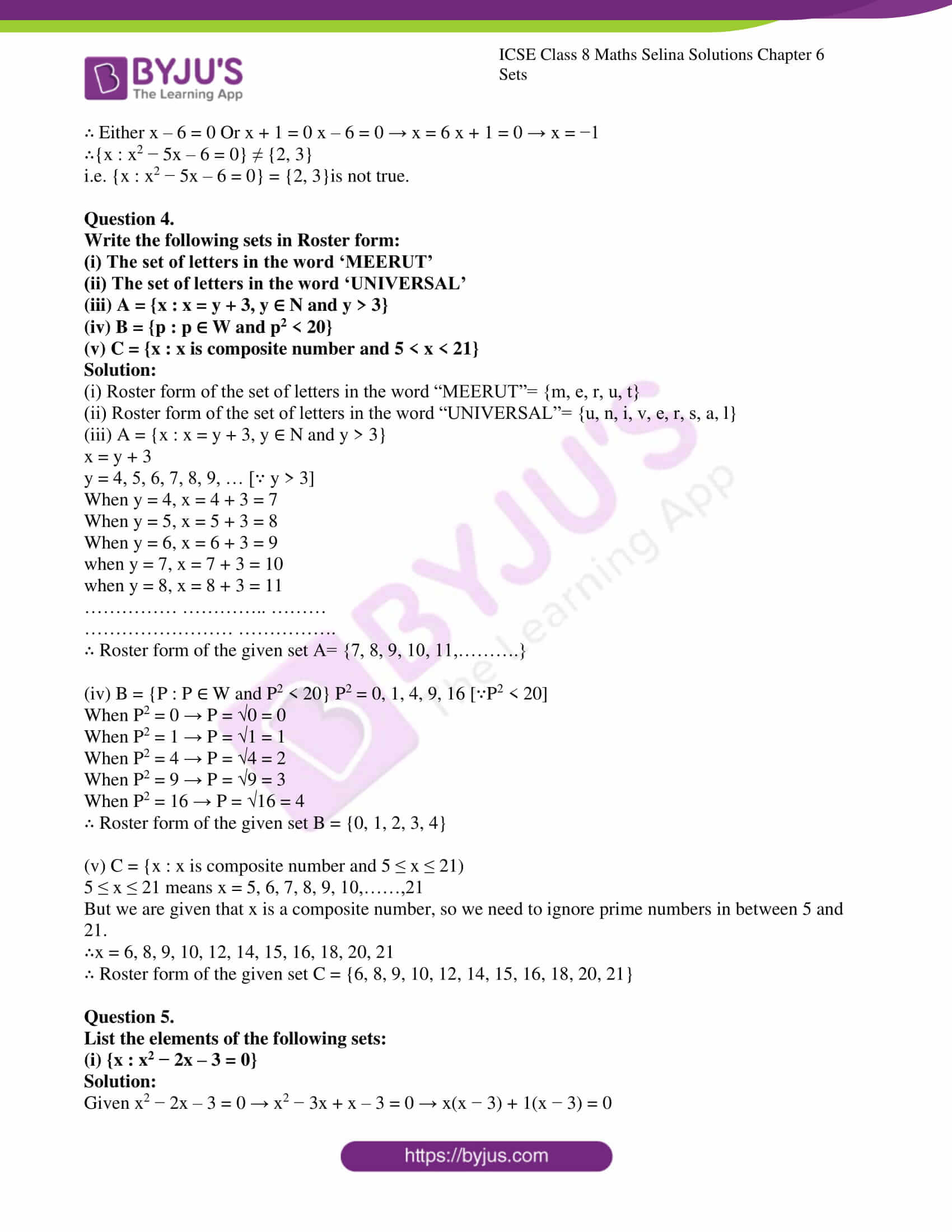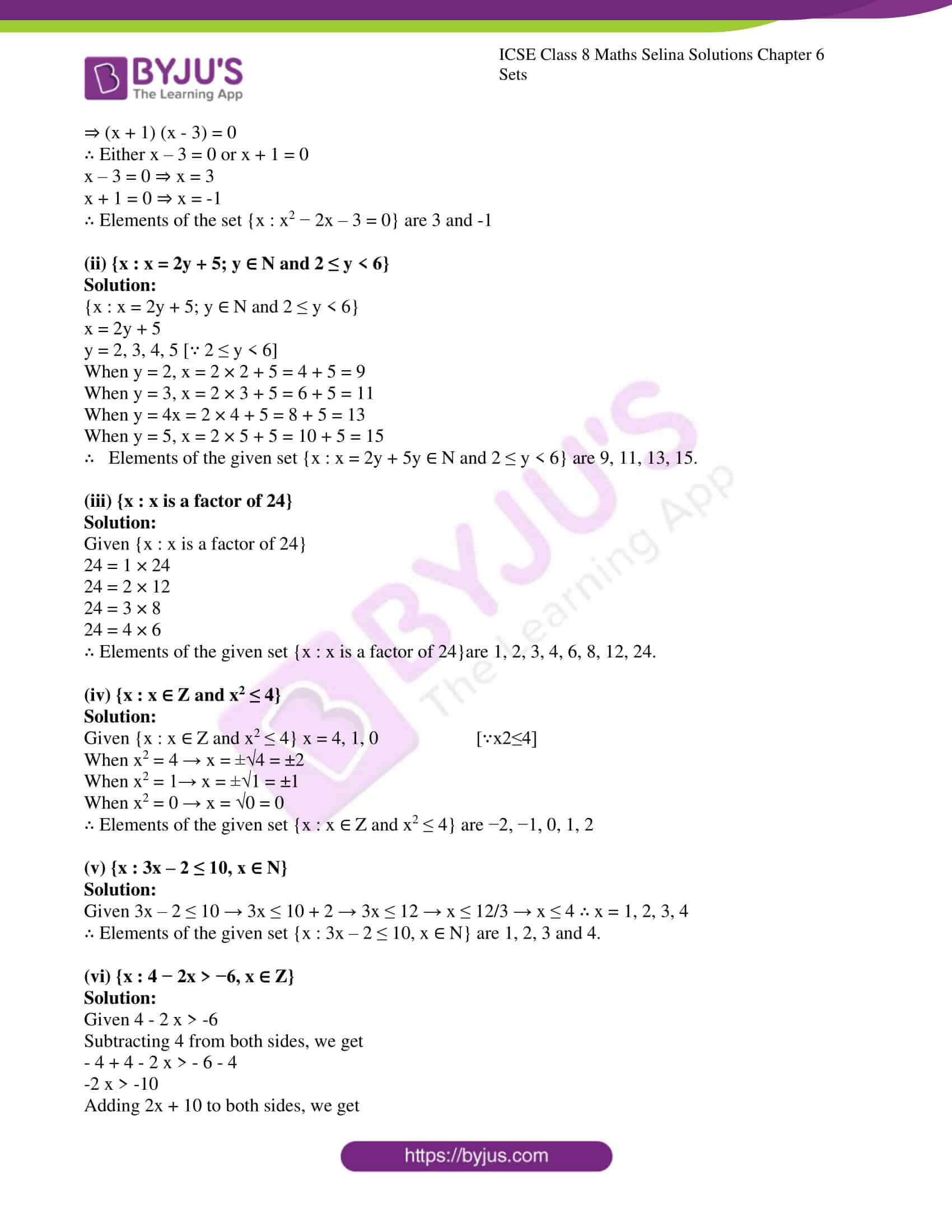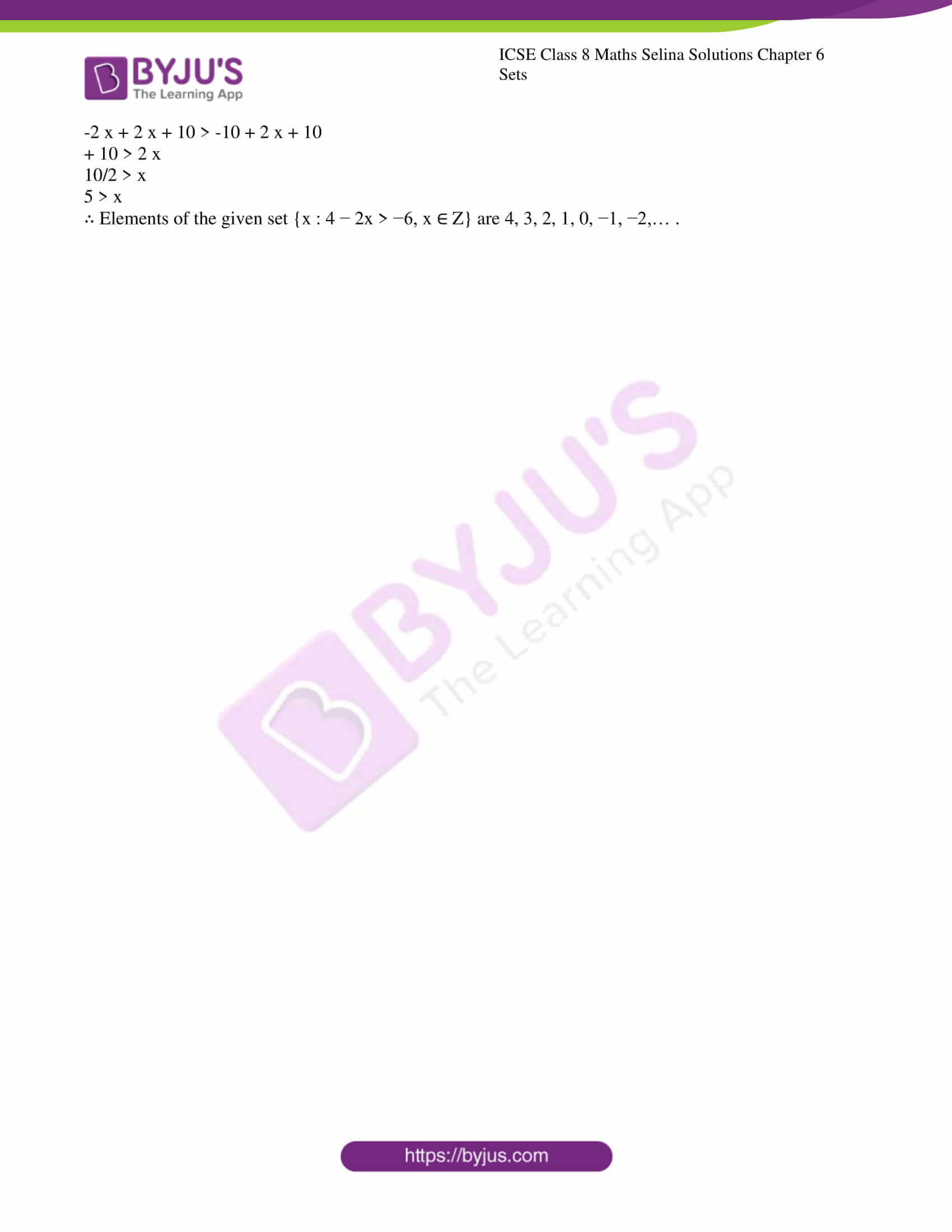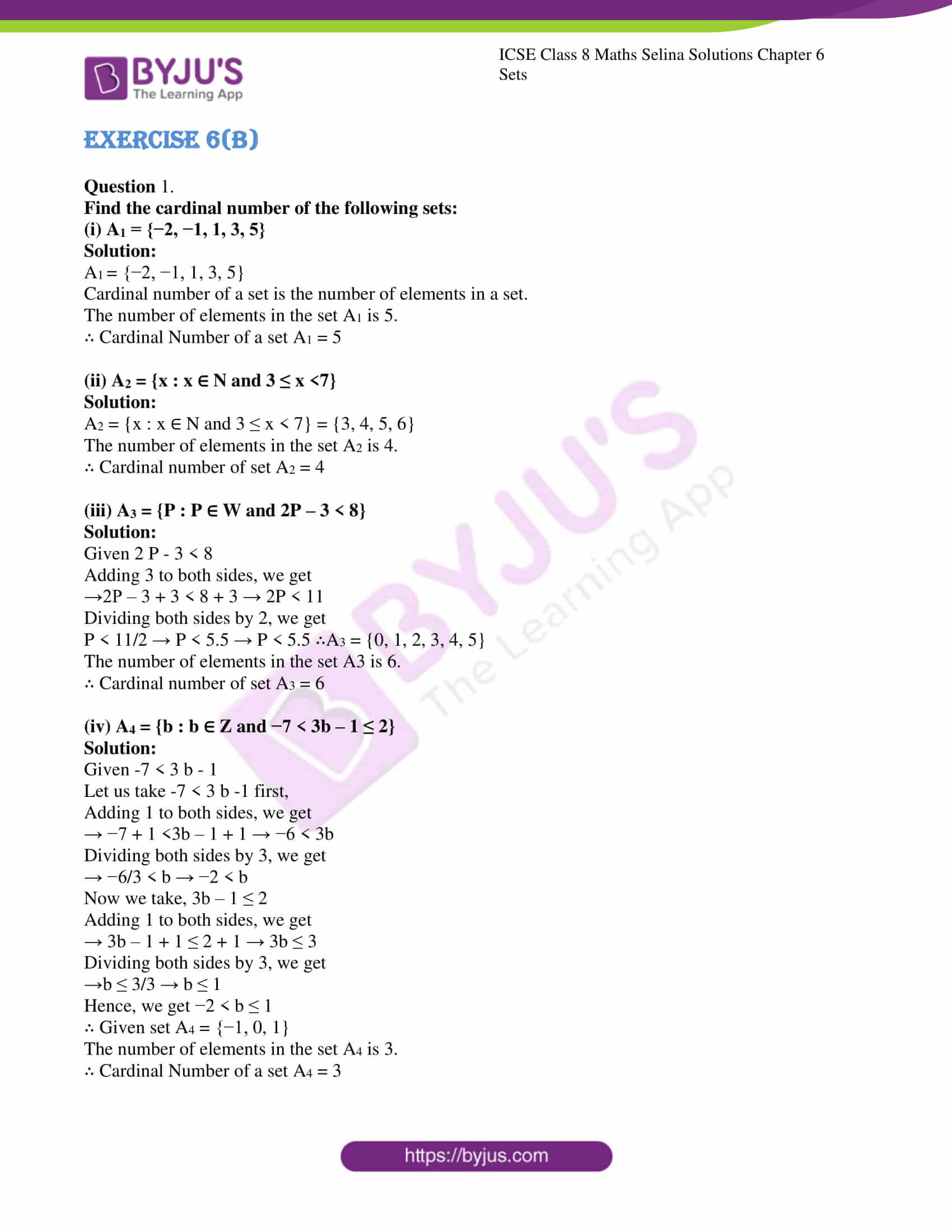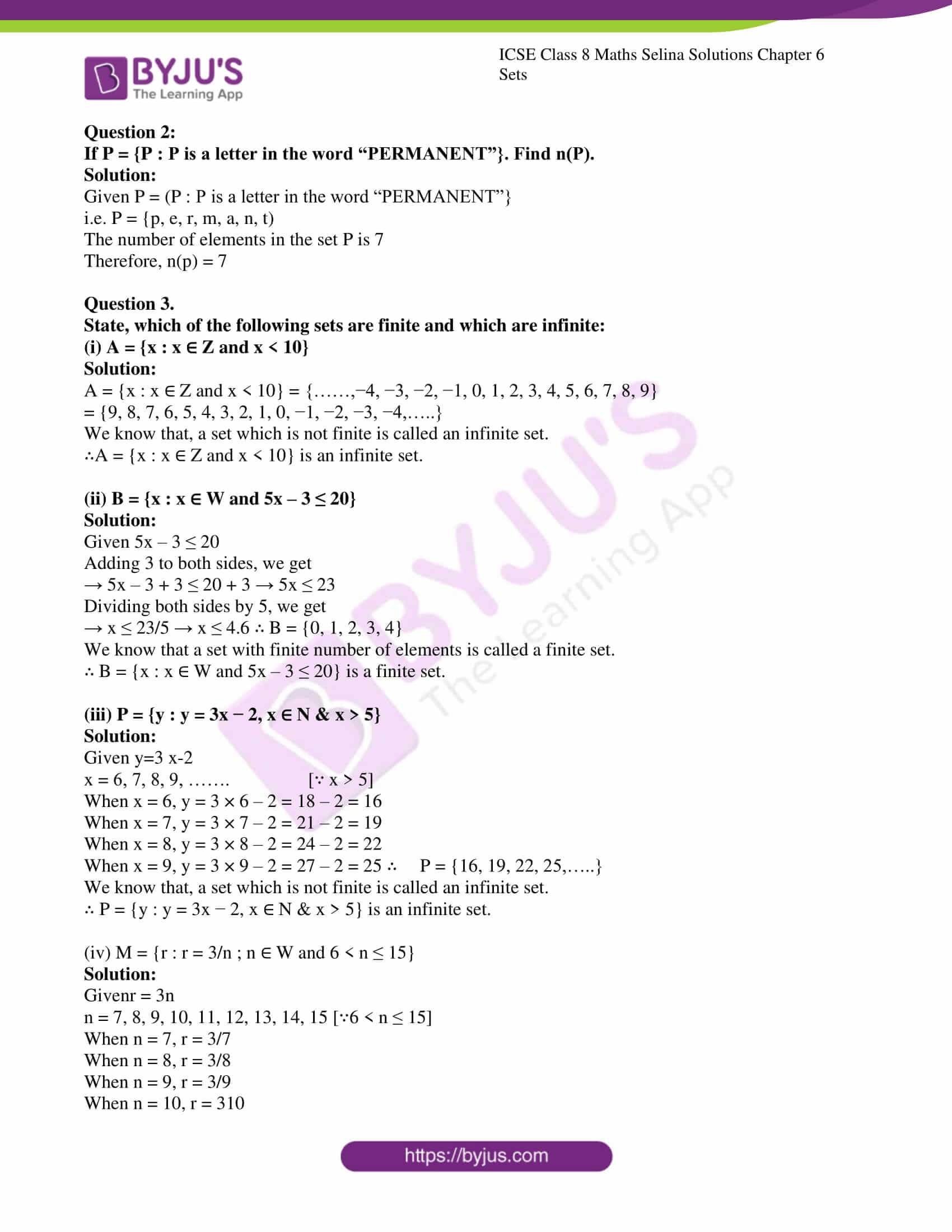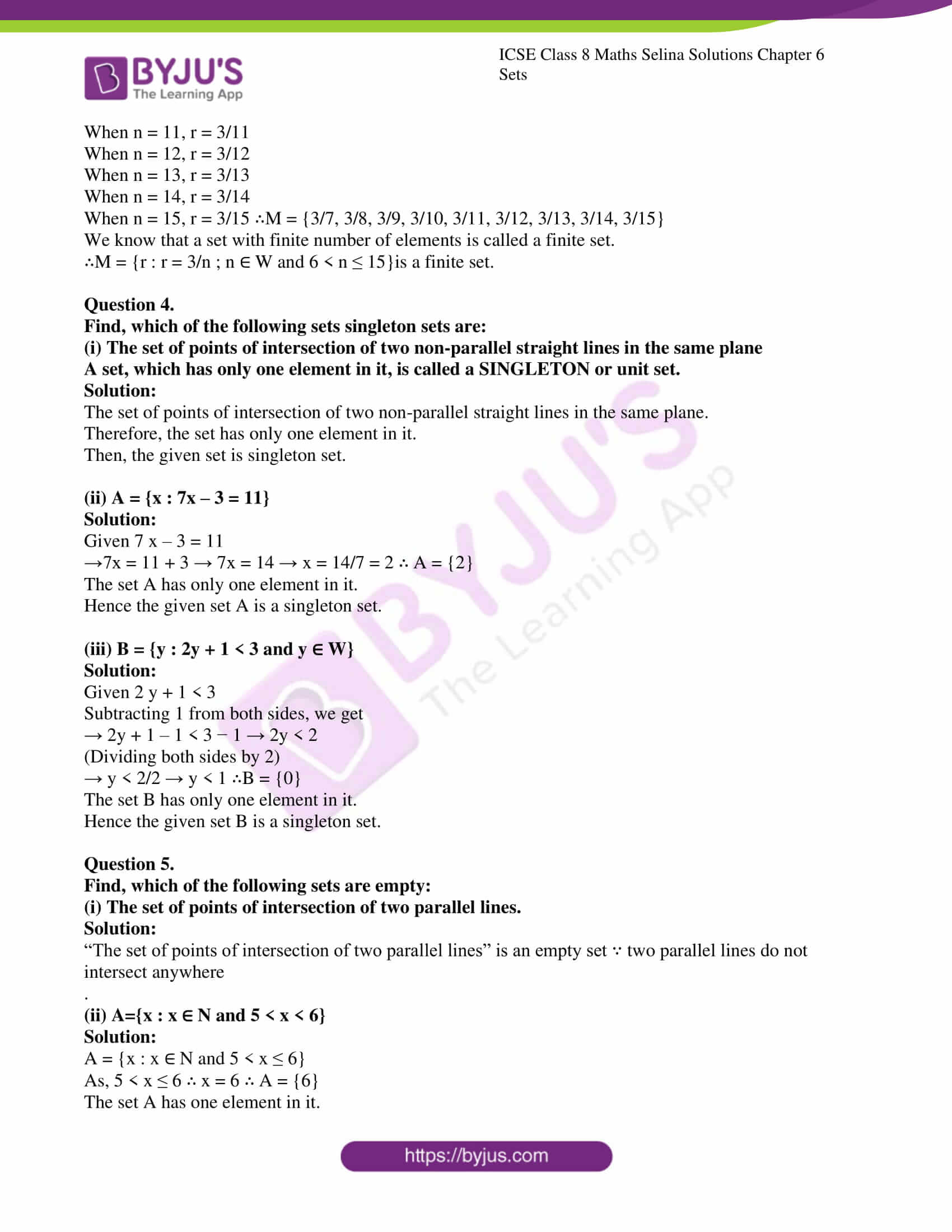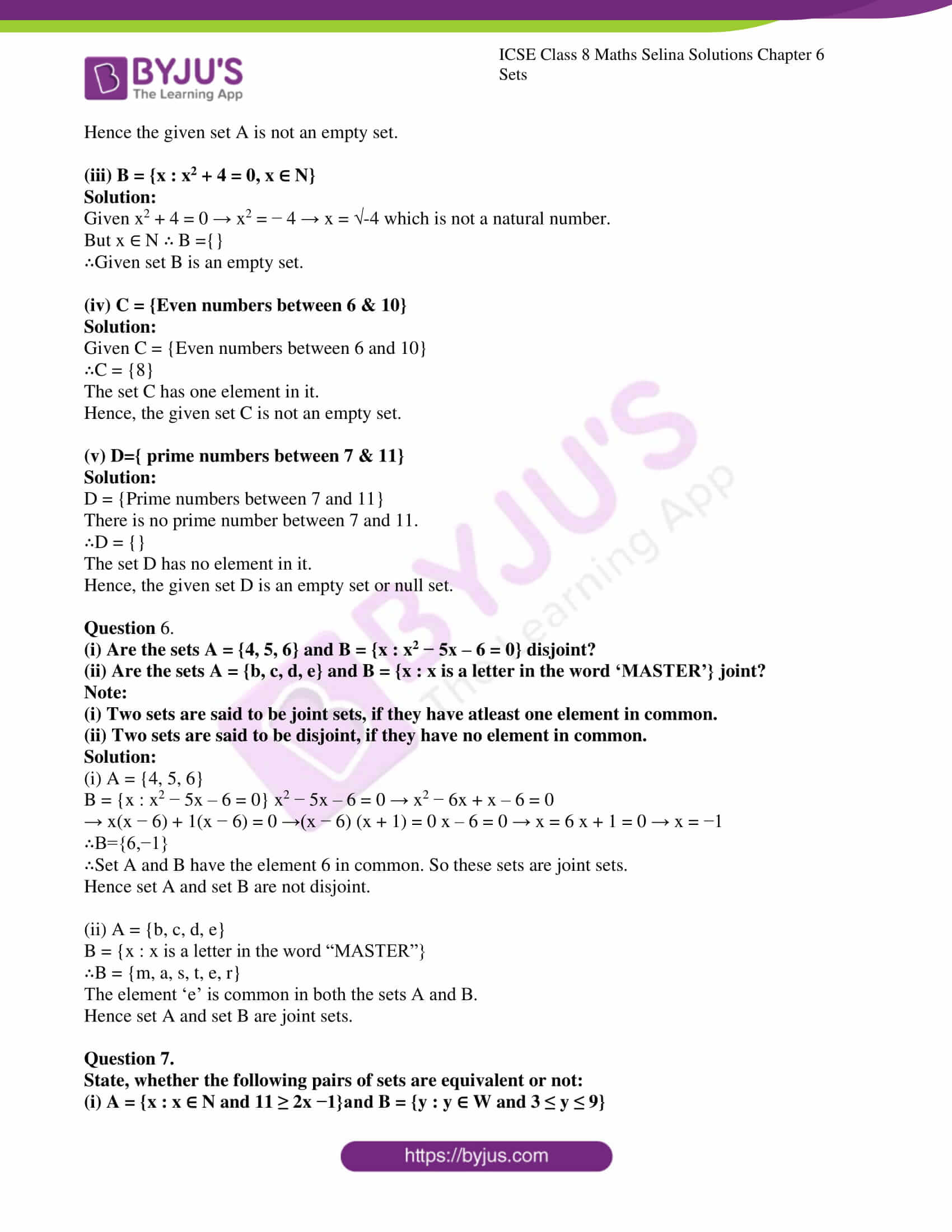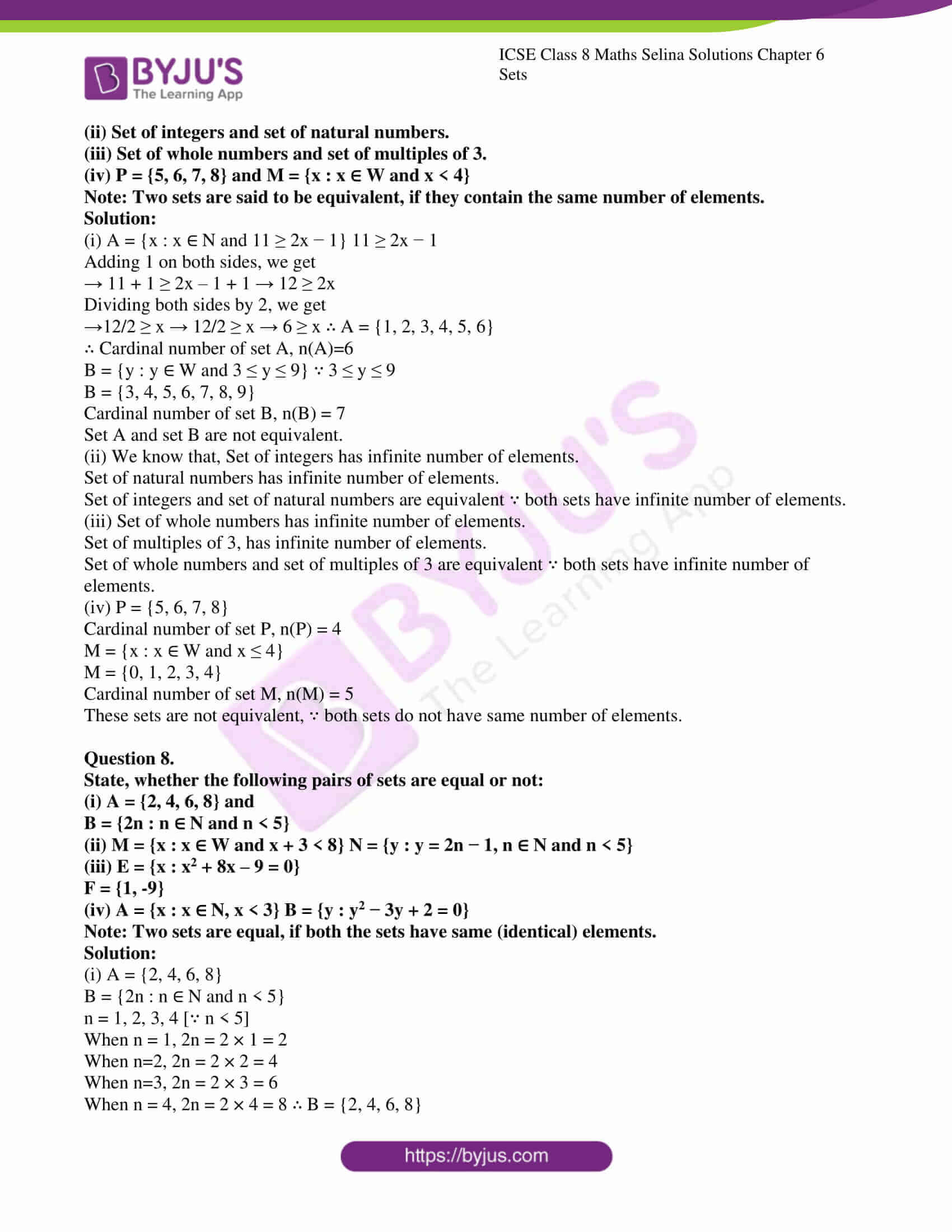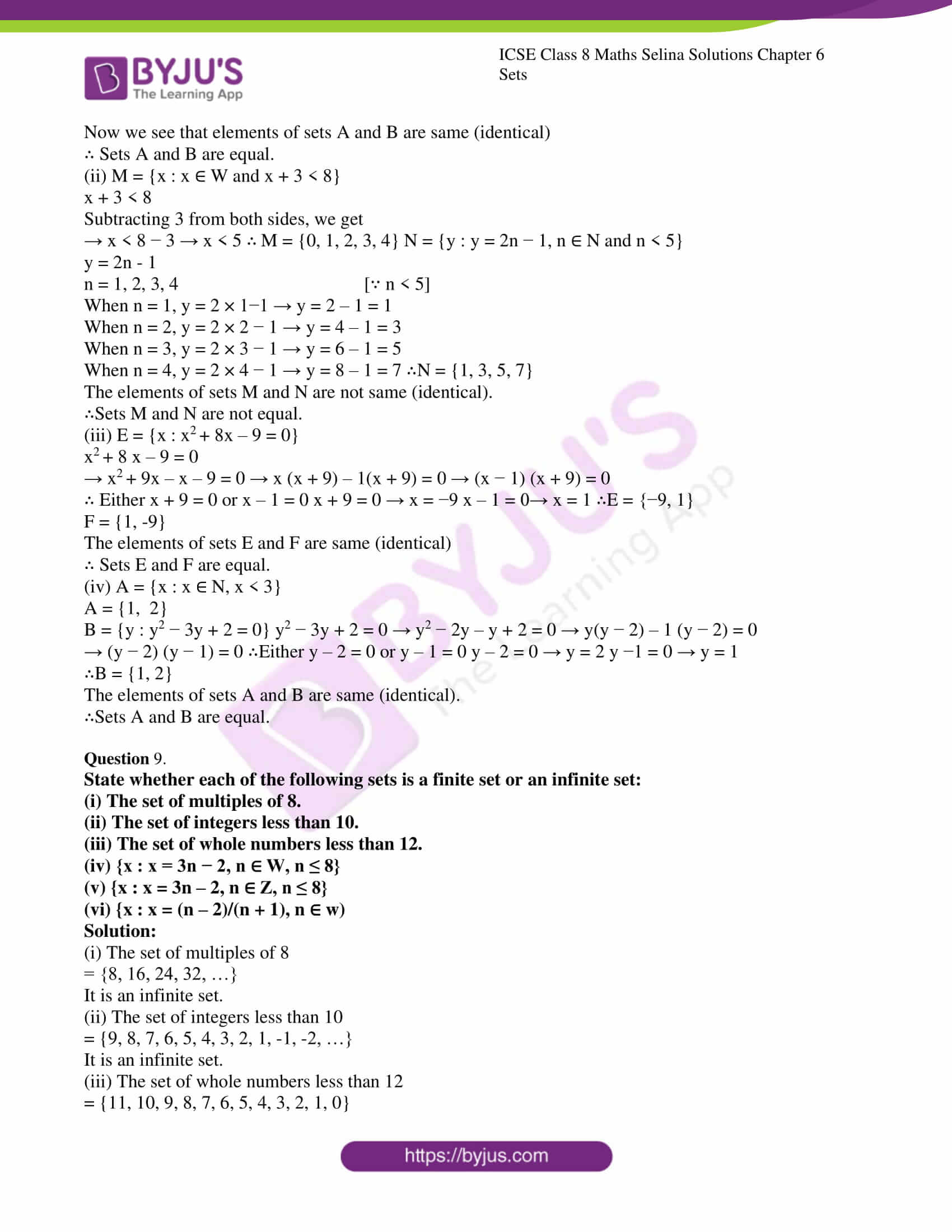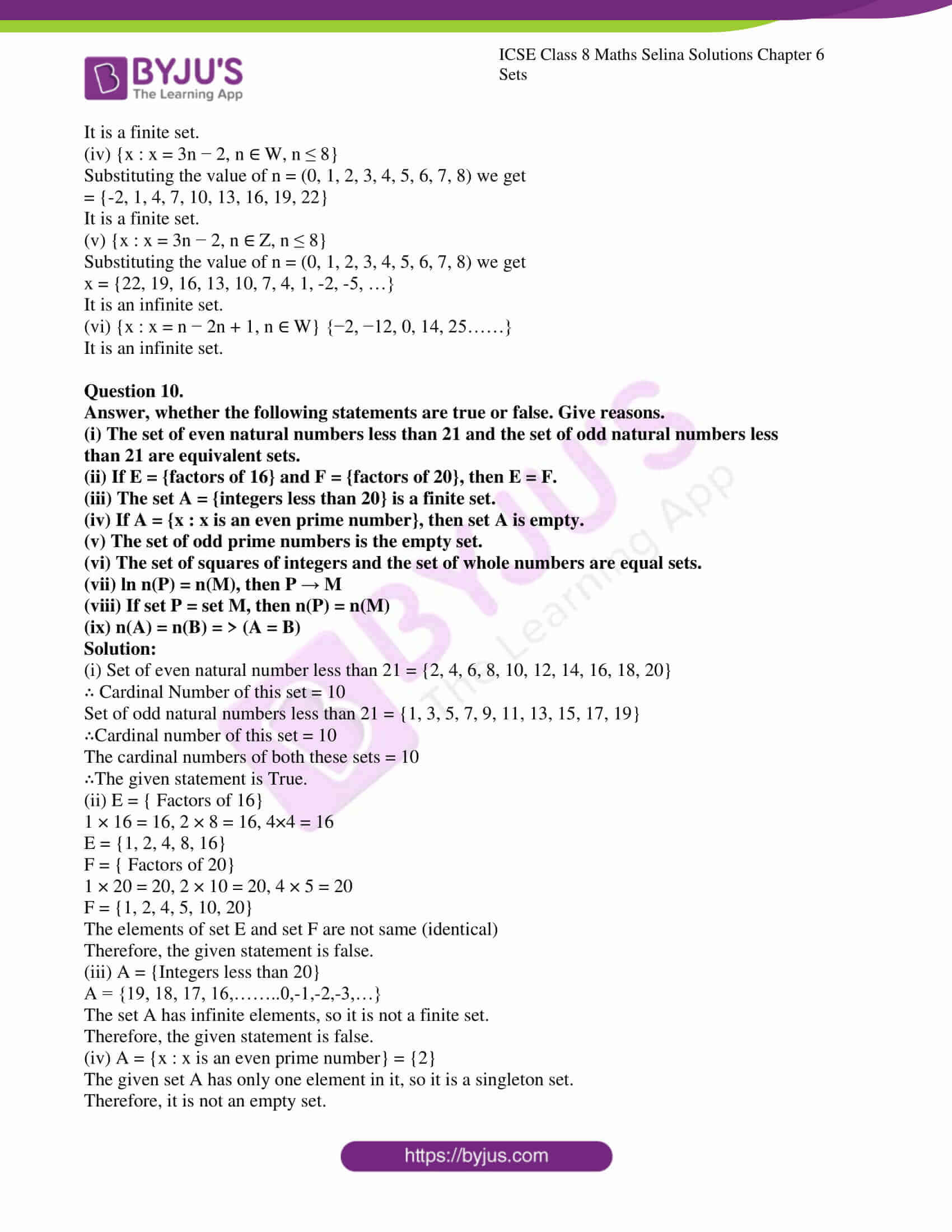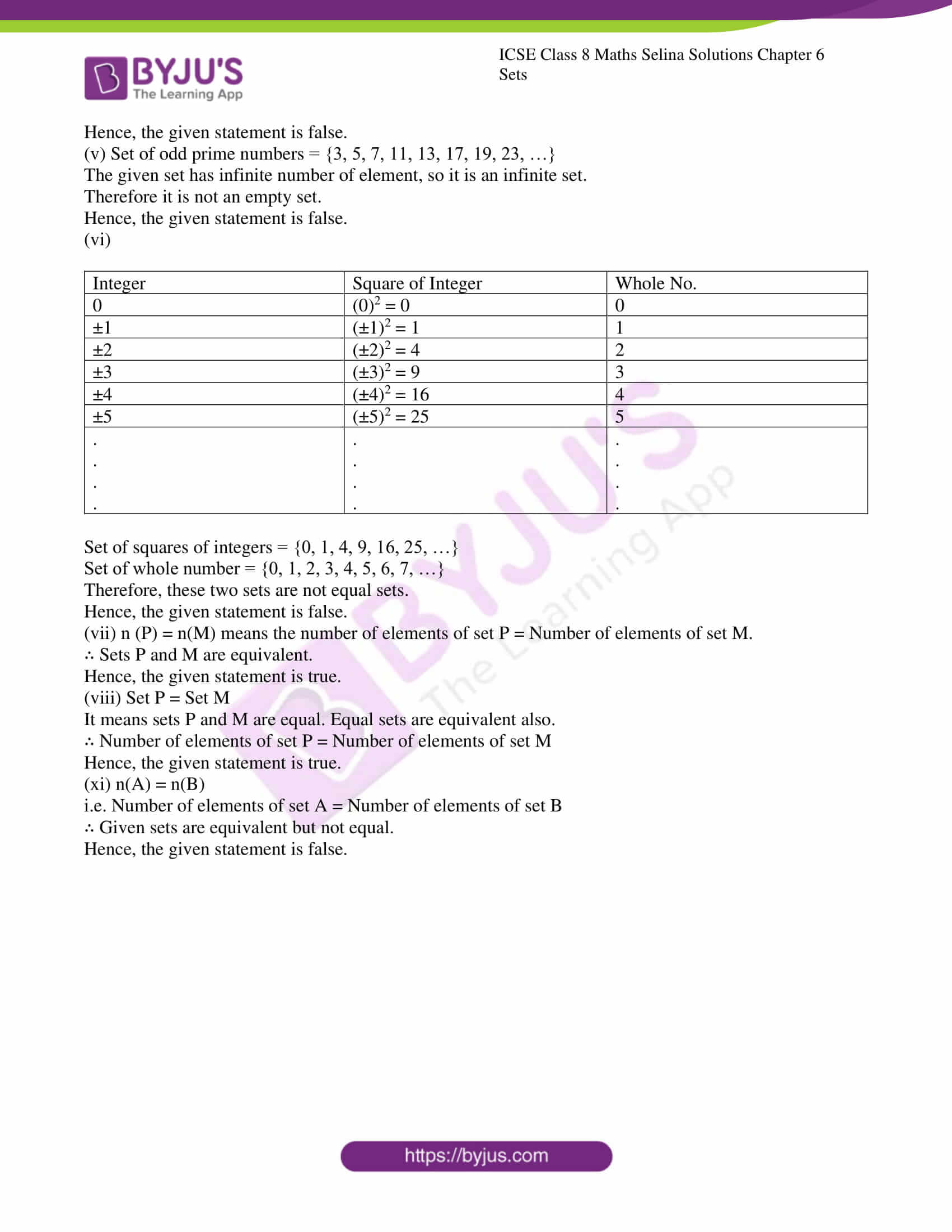ICSE Class 8 Maths Chapter 6 – Sets has two exercises as mentioned below:

1. Exercise 6 (A): It contains a total of 5 questions.
2. Exercise 6 (B): This exercise has a total of 10 questions.

The solutions to all these questions are provided below. Also, students can get the Selina Solutions for all the chapters of class 8 Maths by clicking on ICSE class 8 Maths Selina Solutions page.

### ICSE Class 8 Maths Selina Solutions Chapter 6 Sets – Exercise 6 (A)

Question 1.

Write the following sets in roster (Tabular) form:

(i)

$$\begin{array}{l}A_{1}=\{x : 2 x+3=11\}\end{array}$$

Solution:

∴ 2x+3=11

Shifting the terms 2x=11-3 (Subtracting)

2x=8

$$\begin{array}{l}x=\frac{8}{2} → x=4\end{array}$$

∴ Given set in roster (Tabular) Form is

$$\begin{array}{l}A_{1}=\{4\}\end{array}$$

(ii)

$$\begin{array}{l}A_{2}=\left\{x : x^{2}-4 x-5=0\right\}\end{array}$$

Solution:

Given

$$\begin{array}{l} x^{2}-4 x-5=0\end{array}$$
$$\begin{array}{l}→ x^{2}-5 x+x-5=0\end{array}$$
$$\begin{array}{l}→ x(x-5)+1(x-5)=0\end{array}$$

∴ Either x-5=0 or x+1=0

$$\begin{array}{l}→ \quad x=5 → x=-1\end{array}$$

∴ Given set in roster (Tabular) Form is

$$\begin{array}{l}A_{ 2}=\{5,-1\}\end{array}$$

(iii)

$$\begin{array}{l} A_{3}=\{x : x \in Z,-3 \leq x < 4\}\end{array}$$

Solution:

Given -3≤x < 4

∴ x=-3, -2, -1, 0, 1, 2, 3

∴ Given set in roster (Tabular) form is

$$\begin{array}{l}A_{3}=\{-3,-2,-1,0,1,2,3\}\end{array}$$

(iv)

$$\begin{array}{l} A_{4}={x: is a two digit number and sum of digits of x is 7}\end{array}$$

Solution:

∵ x is a two digit number and sum of digits of x is 7

∴ x=16, 25, 34, 43, 52, 61, 70

∴ Given set in roster (Tabular) form is

$$\begin{array}{l}A_{4}=\{16,25,34,43,52,61,70\}\end{array}$$

(v)

$$\begin{array}{l}\boldsymbol{A}_{5}=\{\boldsymbol{x} : \boldsymbol{x}=\boldsymbol{4} \boldsymbol{n}, \boldsymbol{n} \in \boldsymbol{W} \text { and } \boldsymbol{n} < \mathbf{4}\}\end{array}$$

Solution:

Given x=4n

When n=0, x=4×0

⇒ x=0

n=1, x=4×1=4

⇒ x=4

n=2, x=4×2=8

⇒ x=8

n=3, x=4×3

⇒ x=12

∴ Given set in roster (Tabular) form is

$$\begin{array}{l}A_{5}=\{0,4,8,12\}\end{array}$$

(vi)

$$\begin{array}{l}\mathbf{A}_{6}=\left\{\boldsymbol{x} : \boldsymbol{x}=\frac{\boldsymbol{n}}{n+2} ; \boldsymbol{n} \in \mathbf{N} \& \boldsymbol{n} > \mathbf{5}\right\}\end{array}$$

Solution:

Given

$$\begin{array}{l}x=\frac{n}{n+2}\end{array}$$

When n=6,

$$\begin{array}{l}x=\frac{6}{6+2}\end{array}$$
[∵ n > 5]
$$\begin{array}{l}→ \quad x=\frac{6}{8} → x=\frac{3}{4} \end{array}$$

When,

$$\begin{array}{l}n=7, x=\frac{7}{7+2} → x=\frac{7}{9}\end{array}$$

When,

$$\begin{array}{l}n=8, x=\frac{8}{8+2} → x=\frac{8}{10}\end{array}$$
$$\begin{array}{l}→ \quad x=\frac{4}{5}\end{array}$$

When,

$$\begin{array}{l}n=9, x=\frac{9}{9+2} → x=\frac{9}{11}\end{array}$$

∴ Given set in roster (Tabular) form is

$$\begin{array}{l}\mathrm{A}_{6}=\left\{\frac{3}{4}, \frac{7}{9}, \frac{4}{5}, \frac{9}{11}, \ldots\right\}\end{array}$$

Question 2.

Write the following sets in set-builder (Rule Method) form:

(i)

$$\begin{array}{l}B_{1}=\{6,9,12,15, \dots\} \end{array}$$

Solution:

$$\begin{array}{l}{x:x=3n+3;n∈N} \end{array}$$

(ii)

$$\begin{array}{l}B_{2}=\{11,13,17,19\}\end{array}$$

Solution:

{x: x is a prime number between 10 and 20}

(iii)

$$\begin{array}{l}\mathrm{B}_{3}=\left\{\frac{1}{3}, \frac{3}{5}, \frac{5}{7}, \frac{7}{9}, \frac{9}{11}, \ldots\right\}\end{array}$$

Solution:

$$\begin{array}{l}\left\{x : x=\frac{n}{n+2}, \text { where } n \text { is an odd natural number }\right\}\end{array}$$

(iv)

$$\begin{array}{l} B_{4}=\{8,27,64,125,216\}\end{array}$$

Solution:

$$\begin{array}{l}=\left\{x : x=n^{3} ; n \in N \text { and } 2 \leq n \leq 6\right\}\end{array}$$

(v)

$$\begin{array}{l}B_{5}=\{-5,-4,-3,-2,-1\}\end{array}$$

Solution:

={x:x∈Z,-5≤x≤-1}

(vi)

$$\begin{array}{l}\mathbf{B}_{6}=\{\ldots,-6,-3,0,3,6, \dots\}\end{array}$$

Solution:

={x:x=3n,n∈Z}

Question 3.

(i) Is {1, 2, 4, 16, 64} = {x : x is a factor of 32} ? Give reason.

Solution:

No, {1, 2, 4, 16, 64}≠{x : x is a factor of 32}.

Because 64 is not a factor of 32.

(ii) ls {x : x is a factor of 27}≠{3,9,27,54}? Give reason.

Solution:

Yes, {x:x is a factor of 27}≠{3, 9, 27, 54}

Because 54 is not a factor of 27

(iii) Write the set of even factors of 124.

Solution:

$$\begin{array}{l}1 \times 124=124\end{array}$$

$$\begin{array}{l}2 \times 62=124\end{array}$$

$$\begin{array}{l}4 \times 31=124\end{array}$$

Factors of 124=1, 2, 4, 31, 62, 124

Set of even factors of 124={2, 4, 62, 124}

(iv) Write the set of odd factors of 72.

Solution:

$$\begin{array}{l}1 \times 72=72\end{array}$$

$$\begin{array}{l}2 \times 36=72\end{array}$$

$$\begin{array}{l}3 \times 24=72\end{array}$$

$$\begin{array}{l}4 \times 18=72\end{array}$$

$$\begin{array}{l}6 \times 12=72\end{array}$$

$$\begin{array}{l}8 \times 9=72\end{array}$$

Factors of 72=1, 2, 3, 4, 6, 8, 9, 12, 18, 24, 36, 72

Set of odd factors of 72 = {1, 3, 9}

(v) Write the set of prime factors of 3234.

Solution: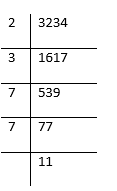$$\begin{array}{l}3234=2 \times 3 \times 7 \times 7 \times 11\end{array}$$

∴ Set of prime factors of 3234={2, 3, 7, 11}

(vi)

$$\begin{array}{l}{ls}\left\{x : x^{2}-7 x+12=0\right\}=\{3,4\} \end{array}$$
?

Solution:

$$\begin{array}{l}x^{2}-7 x+12=0\end{array}$$
$$\begin{array}{l}→ x^{2}-4 x-3 x+12=0\end{array}$$
$$\begin{array}{l}→ x(x-4)-3(x-4)=0\end{array}$$
$$\begin{array}{l}→(x-4)(x-3)=0\end{array}$$

∴   Either x-4=0   or x-3=0

x-4=0   ⇒  x=4

x-3=0⇒  x=3

$$\begin{array}{l}∴\left\{x : x^{2}-7 x+12=0\right\}=\{3,4\}\end{array}$$
, is true.

(vii)

$$\begin{array}{l}1s\left\{x : x^{2}-5 x-6=0\right\}=\{2,3\} \end{array}$$
?

Solution:

$$\begin{array}{l}x^{2}-5 x-6=0\end{array}$$
$$\begin{array}{l}→ x^{2}-6 x+x-6=0\end{array}$$
$$\begin{array}{l}\Rightarrow x(x-6)+1(x-6)=0\end{array}$$
$$\begin{array}{l}\Rightarrow(x-6)(x+1)=0\end{array}$$
$$\begin{array}{l}∴ \quad \text { Either } x-6=0 \quad \text { Or } x+1=0\end{array}$$
$$\begin{array}{l}x-6=0 → x=6\end{array}$$
$$\begin{array}{l}x+1=0 → x=-1\end{array}$$
$$\begin{array}{l}∴\left\{x : x^{2}-5 x-6=0\right\} \neq\{2,3\}\end{array}$$

i.e.

$$\begin{array}{l}\left\{x : x^{2}-5 x-6=0\right\}=\{2,3\} \end{array}$$
is not true.

Question 4.

Write the following sets in Roster form:

(i) The set of letters in the word ‘MEERUT’

(ii) The set of letters in the word ‘UNIVERSAL’

(iii)

$$\begin{array}{l}\boldsymbol{A}=\{\boldsymbol{x} : \boldsymbol{x}=\boldsymbol{y}+\mathbf{3}, \boldsymbol{y} \in \boldsymbol{N} \text { and } \boldsymbol{y} > \mathbf{3}\}\end{array}$$

(iv)

$$\begin{array}{l}\boldsymbol{B}=\left\{\boldsymbol{p} : \boldsymbol{p} \in \boldsymbol{W} \text { and } \boldsymbol{p}^{2} < 20\right\}\end{array}$$

(v) C={x:xis composite number and 5 < x < 21}

Solution:

(i) Roster form of the set of letters in the word “MEERUT”= {m, e, r, u, t}

(ii) Roster form of the set of letters in the word “UNIVERSAL”= {u, n, i, v, e, r, s, a, l}

(iii)

$$\begin{array}{l} A=\{x : x=y+3, y \in N \text { and } y > 3\}\end{array}$$

x=y+3

y=4, 5, 6, 7, 8, 9, … [∵ y > 3]

When y=4,  x=4+3=7

When y=5,  x=5+3=8

When y=6,  x=6+3=9

when y=7,  x=7+3=10

when y=8,  x=8+3=11

…………… ………….. ………

…………………… …………….

∴ Roster form of the given set A= {7, 8, 9, 10, 11,……….}

(iv)

$$\begin{array}{l}B=\left\{P : P \in W \text { and } P^{2} < 20\right\}\end{array}$$
$$\begin{array}{l}P^{2}=0,1,4,9,16 \quad\left[∵ P^{2} < 20\right] \end{array}$$

When

$$\begin{array}{l}P^{2}=0 → P=\sqrt{0}=0 \end{array}$$

When

$$\begin{array}{l}\mathrm{P}^{2}=1 → P=\sqrt{1}=1 \end{array}$$

When

$$\begin{array}{l}\mathrm{P}^{2}=4 → P=\sqrt{4}=2 \end{array}$$

When

$$\begin{array}{l}\mathrm{P}^{2}=9 → P=\sqrt{9}=3 \end{array}$$

When

$$\begin{array}{l}\mathrm{P}^{2}=16 → P=\sqrt{16}=4 \end{array}$$

∴ Roster form of the given set B = {0, 1, 2, 3, 4}

(v)

$$\begin{array}{l}C=\{x : x \text { is composite number and } 5 \leq x \leq 21) \end{array}$$
$$\begin{array}{l}5 \leq x \leq 21 \text { means } x=5,6,7,8,9,10, \ldots \ldots, 21\end{array}$$

But we are given that x is a composite number, so we need to ignore prime numbers in between 5 and 21.

∴x=6, 8, 9, 10, 12, 14, 15, 16, 18, 20, 21

∴ Roster form of the given set C = {6, 8, 9, 10, 12, 14, 15, 16, 18, 20, 21}

Question 5.

List the elements of the following sets:

(i)

$$\begin{array}{l} \left\{x : x^{2}-2 x-3=0\right\}\end{array}$$

Solution:

Given

$$\begin{array}{l} x^{2}-2 x-3=0\end{array}$$
$$\begin{array}{l}→ x^{2}-3 x+x-3=0\end{array}$$
$$\begin{array}{l}→ x(x-3)+1(x-3)=0\end{array}$$

⇒  (x+1)(x-3)=0

∴   Either x-3=0   or  x+1=0

x-3=0 ⇒ x=3

x+1=0⇒ x=-1

∴   Elements of the set

$$\begin{array}{l} \left\{x : x^{2}-2 x-3=0\right\}\end{array}$$
are 3 and -1

(ii)

$$\begin{array}{l}\{x : x=2 y+5 ; y \in N \text { and } 2 \leq y < 6\}\end{array}$$

Solution:

$$\begin{array}{l}\{x : x=2 y+5 ; y \in \mathrm{N} \text { and } 2 \leq y < 6\}\end{array}$$

x=2y+5

y=2, 3, 4, 5 [∵2≤y < 6]

When

$$\begin{array}{l}y=2, x=2 \times 2+5=4+5=9\end{array}$$

When

$$\begin{array}{l}y=3, x=2 \times 3+5=6+5=11\end{array}$$

When

$$\begin{array}{l}y=4 x=2 \times 4+5=8+5=13\end{array}$$

When

$$\begin{array}{l}y=5, x=2 \times 5+5=10+5=15\end{array}$$

∴   Elements of the given set

$$\begin{array}{l}\{x : x=2 y+5 y \in N \text { and } 2 \leq y < 6\} \end{array}$$
are 9, 11, 13, 15.

(iii)

$$\begin{array}{l}\{x : x \text { is a factor of } 24\} \end{array}$$

Solution:

Given

$$\begin{array}{l}\{x : x \text { is a factor of } 24\}\end{array}$$

$$\begin{array}{l}24=1 \times 24\end{array}$$

$$\begin{array}{l}24=2 \times 12\end{array}$$

$$\begin{array}{l}24=3 \times 8\end{array}$$

$$\begin{array}{l}24=4 \times 6\end{array}$$

∴   Elements of the given set

$$\begin{array}{l}\{x : x \text { is a factor of } 24\} \end{array}$$
are 1, 2, 3, 4, 6, 8, 12, 24.

(iv)

$$\begin{array}{l}\left\{x : x \in Z \text { and } x^{2} \leq 4\right\} \end{array}$$

Solution:

Given

$$\begin{array}{l}\left\{x : x \in Z \text { and } x^{2} \leq 4\right\} \end{array}$$
$$\begin{array}{l}x=4,1,0 \quad\left[∵ x^{2} \leq 4\right] \end{array}$$

When

$$\begin{array}{l}x^{2}=4 → x=\pm \sqrt{4}=\pm 2 \end{array}$$

When

$$\begin{array}{l}x^{2}=1 → x=\pm \sqrt{1}=\pm 1 \end{array}$$

When

$$\begin{array}{l}x^{2}=0 → x=\sqrt{0}=0 \end{array}$$

∴   Elements of the given set

$$\begin{array}{l}\left\{x : x \in \mathrm{Z} \text { and } x^{2} \leq 4\right\} \text { are }-2,-1,0,1,2\end{array}$$

(v)

$$\begin{array}{l}\{x : 3 x-2 \leq 10, x \in N\} \end{array}$$

Solution:

Given

$$\begin{array}{l}3 x-2 \leq 10 \end{array}$$
$$\begin{array}{l}→ \quad 3 x \leq 10+2\end{array}$$
$$\begin{array}{l}→ \quad 3 x \leq 12\end{array}$$
$$\begin{array}{l}→ \quad x \leq \frac{12}{3}\end{array}$$
$$\begin{array}{l}→ \quad x \leq 4\end{array}$$
$$\begin{array}{l}∴ x=1,2,3,4\end{array}$$

∴   Elements of the given set

$$\begin{array}{l}\{x : 3 x-2 \leq 10, x \in \mathrm{N}\} \text { are } 1,2,3 \text { and } 4\end{array}$$
.

(vi)

$$\begin{array}{l}\{x : 4-2 x > -6, x \in Z\} \end{array}$$

Solution:

Given 4-2 x > -6

Subtracting 4 from both sides, we get

-4+4-2 x > -6-4

-2 x > -10

Adding 2x+10 to both sides, we get

-2 x+2 x+10 > -10+2 x+10

+10 > 2 x

$$\begin{array}{l}\frac{10}{2} > x\end{array}$$

5 > x

∴ Elements of the given set

$$\begin{array}{l}\{x : 4-2 x > -6, x \in \mathrm{Z}\} \text { are } 4,3,2,1,0,-1,-2, \ldots\end{array}$$
.

### ICSE Class 8 Maths Selina Solutions Chapter 6 Sets – Exercise 6 (B)

Question 1.
Find the cardinal number of the following sets:

(i)

$$\begin{array}{l}A_{1}=\{-2,-1,1,3,5\}\end{array}$$

Solution:

$$\begin{array}{l}A_{1}=\{-2,-1,1,3,5\}\end{array}$$

Cardinal number of a set is the number of elements in a set.

The number of elements in the set

$$\begin{array}{l}A_{1} \text { is } 5\end{array}$$
.

∴ Cardinal Number of a set

$$\begin{array}{l}A_{1}=5\end{array}$$

(ii)

$$\begin{array}{l}A_{2}=\{x : x \in N \text { and } 3 \leq x < 7\}\end{array}$$

Solution:

$$\begin{array}{l}A_{2}=\{x : x \in N \text { and } 3 \leq x < 7\}\end{array}$$
$$\begin{array}{l}=\{3,4,5,6\}\end{array}$$

The number of elements in the set

$$\begin{array}{l}A_{2} \end{array}$$
is 4.

∴ Cardinal number of set

$$\begin{array}{l}A_{2}=4\end{array}$$

(iii)

$$\begin{array}{l}A_{3}=\{P : P \in \mathbb{W} \text { and } 2 P-3 < 8\}\end{array}$$

Solution:

Given 2 P-3 < 8

Adding 3 to both sides, we get

$$\begin{array}{l}→ 2 P-3+3 < 8+3\end{array}$$
$$\begin{array}{l}→ \quad 2 \mathrm{P} < 11\end{array}$$

Dividing both sides by 2, we get

$$\begin{array}{l}P < \frac{11}{2}\end{array}$$
$$\begin{array}{l}→ \quad P < 5.5\end{array}$$
$$\begin{array}{l}→ \quad P < 5.5\end{array}$$
$$\begin{array}{l}∴ A_{3}=\{0,1,2,3,4,5\}\end{array}$$

The number of elements in the set

$$\begin{array}{l}A_{3}\end{array}$$
is 6.

∴ Cardinal number of set

$$\begin{array}{l}A_{3}=6 \end{array}$$

(iv)

$$\begin{array}{l}A_{4}=\{b : b \in Z \text { and }-7 < 3 b-1 \leq 2\}\end{array}$$

Solution:

Given -7 < 3 b-1

Let us take -7 < 3 b-1 first,

Adding 1 to both sides, we get

$$\begin{array}{l}→ \quad-7+1 < 3 b-1+1\end{array}$$
$$\begin{array}{l}→ \quad-6 < 3 b\end{array}$$

Dividing both sides by 3, we get

$$\begin{array}{l}→ \quad-\frac{6}{3} < b\end{array}$$
$$\begin{array}{l}→ \quad-2 < b\end{array}$$

Now we take,

$$\begin{array}{l}3 b-1 \leq 2\end{array}$$

Adding 1 to both sides, we get

$$\begin{array}{l}→ \quad 3 b-1+1 \leq 2+1\end{array}$$
$$\begin{array}{l}→ \quad 3 b \leq 3\end{array}$$

Dividing both sides by 3, we get

$$\begin{array}{l}→ b \leq \frac{3}{3}\end{array}$$
$$\begin{array}{l}→ b \leq 1\end{array}$$

Hence, we get

$$\begin{array}{l}-2 < b \leq 1\end{array}$$

∴ Given set

$$\begin{array}{l}A_{4}=\{-1,0,1\}\end{array}$$

The number of elements in the set

$$\begin{array}{l}A_{4} \end{array}$$
is 3.

∴ Cardinal Number of a set

$$\begin{array}{l}A_{4}=3\end{array}$$

Question 2:

If P={P:P is a letter in the word “PERMANENT”}. Find n(P).

Solution:

Given P=(P:P is a letter in the word “PERMANENT”}

I.e. P = {p, e, r, m, a, n, t)

The number of elements in the set P is 7

Therefore, n(p) =7

Question 3.

State, which of the following sets are finite and which are infinite:

(i)

$$\begin{array}{l}A=\{x : x \in Z \text { and } x < 10\}\end{array}$$

Solution:

$$\begin{array}{l}A=\{x : x \in Z \text { and } x < 10\}\end{array}$$
$$\begin{array}{l}=\{\ldots \ldots,-4,-3,-2,-1,0,1,2,3,4,5,6,7,8,9\}\end{array}$$
$$\begin{array}{l}=\{9,8,7,6,5,4,3,2,1,0,-1,-2,-3,-4, \ldots . .\} \end{array}$$

We know that, a set which is not finite is called an infinite set.

$$\begin{array}{l}∴ A=\{x : x \in Z \text { and } x < 10\} \end{array}$$
is an infinite set.

(ii)

$$\begin{array}{l}B=\{x : x \in W \text { and } 5 x-3 \leq 20\}\end{array}$$

Solution:

Given

$$\begin{array}{l}5 x-3 \leq 20 \end{array}$$

Adding 3 to both sides, we get

$$\begin{array}{l}→ 5 x-3+3 \leq 20+3\end{array}$$
$$\begin{array}{l}→ \quad 5 x \leq 23\end{array}$$

Dividing both sides by 5, we get

$$\begin{array}{l}→ x \leq \frac{23}{5}\end{array}$$
$$\begin{array}{l}→ x \leq 4.6\end{array}$$
$$\begin{array}{l}∴ \quad B=\{0,1,2,3,4\}\end{array}$$

We know that a set with finite number of elements is called a finite set.

$$\begin{array}{l}∴ \quad B=\{x : x \in W \text { and } 5 x-3 \leq 20\} \end{array}$$
is a finite set.

(iii)

$$\begin{array}{l} \mathrm{P}=\{y : y=3 x-2, x \in \mathrm{N} \& x > 5\}\end{array}$$

Solution:

Given y=3 x-2

x=6, 7, 8, 9, …….

$$\begin{array}{l} [∵ x > 5] \end{array}$$

When

$$\begin{array}{l}x=6, y=3 \times 6-2=18-2=16\end{array}$$

When

$$\begin{array}{l}x=7, \quad y=3 \times 7-2=21-2=19 \end{array}$$

When

$$\begin{array}{l}x=8, y=3 \times 8-2=24-2=22 \end{array}$$

When

$$\begin{array}{l}x=9, \quad y=3 \times 9-2=27-2=25 \end{array}$$
$$\begin{array}{l}∴ \quad \mathrm{P}=\{16,19,22,25, \ldots . .\} \end{array}$$

We know that, a set which is not finite is called an infinite set.

∴

$$\begin{array}{l} \mathrm{P}=\{y : y=3 x-2, x \in \mathrm{N} \& x > 5\}\end{array}$$
is an infinite set.

(iv)

$$\begin{array}{l}\mathrm{M}=\left\{r : r=\frac{3}{n} ; n \in \mathrm{W} \text { and } 6 < n \leq 15\right\}\end{array}$$

Solution:

Given

$$\begin{array}{l} r=\frac{3}{n}\end{array}$$

n=7, 8, 9, 10, 11, 12, 13, 14, 15

$$\begin{array}{l}[∵ 6 < n \leq 15] \end{array}$$

When

$$\begin{array}{l}n=7, r=\frac{3}{7} \end{array}$$

When

$$\begin{array}{l}n=8, r=\frac{3}{8} \end{array}$$

When

$$\begin{array}{l}n=9, \quad r=\frac{3}{9} \end{array}$$

When

$$\begin{array}{l}n=10, r=\frac{3}{10} \end{array}$$

When

$$\begin{array}{l}n=11, \quad r=\frac{3}{11} \end{array}$$

When

$$\begin{array}{l}n=12, r=\frac{3}{12} \end{array}$$

When

$$\begin{array}{l}n=13, r=\frac{3}{13} \end{array}$$

When

$$\begin{array}{l}n=14, r=\frac{3}{14} \end{array}$$

When

$$\begin{array}{l}n=15, r=\frac{3}{15} \end{array}$$
$$\begin{array}{l}∴ \quad \mathrm{M}=\left\{\frac{3}{7}, \frac{3}{8}, \frac{3}{9}, \frac{3}{10}, \frac{3}{11}, \frac{3}{12}, \frac{3}{13}, \frac{3}{14}, \frac{3}{15}\right\}\end{array}$$

We know that a set with finite number of elements is called a finite set.

$$\begin{array}{l}∴ \mathrm{M}=\left\{r : r=\frac{3}{n} ; n \in \mathrm{W} \text { and } 6 < n \leq 15\right\} \end{array}$$
is a finite set.

Question 4.

Find, which of the following sets singleton sets are:

(i) The set of points of intersection of two non-parallel straight lines in the same plane

A set, which has only one element in it, is called a SINGLETON or unit set.

Solution:

The set of points of intersection of two non-parallel straight lines in the same plane.

Therefore, the set has only one element in it.

Then, the given set is singleton set.

(ii)

$$\begin{array}{l}A=\{x : 7 x-3=11\} \end{array}$$

Solution:

Given 7 x-3=11

$$\begin{array}{l}→ \quad 7 x=11+3\end{array}$$
$$\begin{array}{l}→ \quad 7 x=14\end{array}$$
$$\begin{array}{l}→ \quad x=\frac{14}{7}=2\end{array}$$
$$\begin{array}{l}∴ \quad A=\{2\}\end{array}$$

The set A has only one element in it.

Hence the given set A is a singleton set.

(iii)

$$\begin{array}{l}B=\{y : 2 y+1 < 3 \text { and } y \in W\}\end{array}$$

Solution:

Given 2 y+1 < 3

Subtracting 1 from both sides, we get

$$\begin{array}{l}→ 2 y+1-1 < 3-1\end{array}$$
$$\begin{array}{l}→ 2 y < 2\end{array}$$

(Dividing both sides by 2)

$$\begin{array}{l}→ y < \frac{2}{2}\end{array}$$
$$\begin{array}{l}→ y < 1\end{array}$$
$$\begin{array}{l}∴ \quad B=\{0\}\end{array}$$

The set B has only one element in it.

Hence the given set B is a singleton set.

Question 5.

Find, which of the following sets are empty:

(i) The set of points of intersection of two parallel lines.

Solution:

“The set of points of intersection of two parallel lines” is an empty set ∵ two parallel lines do not intersect anywhere.

(ii)

$$\begin{array}{l}\boldsymbol{A}=\{\boldsymbol{x} : \boldsymbol{x} \in \boldsymbol{N} \text { and } 5 < \boldsymbol{x} < 6\}\end{array}$$

Solution:

$$\begin{array}{l}A=\{x : x \in \mathrm{N} \text { and } 5 < x \leq 6\}\end{array}$$

As,

$$\begin{array}{l}5 < x \leq 6\end{array}$$
$$\begin{array}{l}∴ \quad x=6\end{array}$$
$$\begin{array}{l}∴ A=\{6\}\end{array}$$

The set A has one element in it.

Hence the given set A is not an empty set.

(iii)

$$\begin{array}{l} B=\left\{x : x^{2}+4=0, x \in N\right\}\end{array}$$

Solution:

Given

$$\begin{array}{l}x^{2}+4=0 \end{array}$$
$$\begin{array}{l}→ \quad x^{2}=-4\end{array}$$
$$\begin{array}{l}→ \quad x=\sqrt{-4}\end{array}$$
which is not a natural number.

But

$$\begin{array}{l}x \in \mathbf{N} \end{array}$$
$$\begin{array}{l}∴ \quad \mathbf{B}=\{ \}\end{array}$$

∴Given set B is an empty set.

(iv)

$$\begin{array}{l}C=\{\text { Even numbers between } 6 \& 10\} \end{array}$$

Solution:

Given C = {Even numbers between 6 and 10}

$$\begin{array}{l}∴ C=\{8\}\end{array}$$

The set C has one element in it.

Hence, the given set C is not an empty set.

(v) D={ prime numbers between 7 & 11}

Solution:

D={Prime numbers between 7 and 11}

There is no prime number between 7 and 11.

$$\begin{array}{l}∴ \mathrm{D}=\{ \}\end{array}$$

The set D has no element in it.

Hence, the given set D is an empty set or null set.

Question 6.

(i) Are the sets A = {4, 5, 6} and

$$\begin{array}{l}B=\left\{x : x^{2}-5 x-6=0\right\}\end{array}$$
disjoint?

(ii) Are the sets A={b,c,d,e} and B={x:x is a letter in the word ‘MASTER’} joint?

Note:

(i) Two sets are said to be joint sets, if they have atleast one element in common.

(ii) Two sets are said to be disjoint, if they have no element in common.

Solution:

(i) A={4,5,6}

$$\begin{array}{l}B=\left\{x : x^{2}-5 x-6=0\right\}\end{array}$$
$$\begin{array}{l}x^{2}-5 x-6=0\end{array}$$
$$\begin{array}{l}→ x^{2}-6 x+x-6=0\end{array}$$
$$\begin{array}{l}→ x(x-6)+1(x-6)=0\end{array}$$
$$\begin{array}{l}→(x-6)(x+1)=0\end{array}$$
$$\begin{array}{l}x-6=0 → x=6\end{array}$$
$$\begin{array}{l}x+1=0 → x=-1\end{array}$$
$$\begin{array}{l}∴ \quad B=\{6,-1\}\end{array}$$

∴Set A and B have the element 6 in common. So these sets are joint sets.

Hence set A and set B are not disjoint.

(ii) A={b,c,d,e}

B={x:x is a letter in the word “MASTER”}

$$\begin{array}{l}∴ \quad \mathrm{B}=\{m, a, s, t, e, r\}\end{array}$$

The element ‘e’ is common in both the sets A and B.

Hence set A and set B are joint sets.

Question 7.

State, whether the following pairs of sets are equivalent or not:

(i)

$$\begin{array}{l}A=\{x : x \in N \text { and } 11 \geq 2 x-1\} and B=\{y : y \in W \text { and } 3 \leq y \leq 9\}\end{array}$$

(ii) Set of integers and set of natural numbers.

(iii) Set of whole numbers and set of multiples of 3.

(iv) P={5,6,7,8} and

$$\begin{array}{l}M=\{x : x \in W \text { and } x < 4\} \end{array}$$

Note: Two sets are said to be equivalent, if they contain the same number of elements.

Solution:

(i)

$$\begin{array}{l}\mathrm{A}=\{x : x \in \mathrm{N} \text { and } 11 \geq 2 x-1\} \end{array}$$
$$\begin{array}{l}11 \geq 2 x-1\end{array}$$

Adding 1 on both sides, we get

$$\begin{array}{l}→ 11+1 \geq 2 x-1+1 \end{array}$$
$$\begin{array}{l}→ \quad 12 \geq 2 x\end{array}$$

Dividing both sides by 2, we get

$$\begin{array}{l}→ \quad \frac{12}{2} \geq x\end{array}$$
$$\begin{array}{l}→ \quad \frac{12}{2} \geq x\end{array}$$
$$\begin{array}{l}→ 6 \geq x\end{array}$$
$$\begin{array}{l}∴ A=\{1,2,3,4,5,6\}\end{array}$$

∴ Cardinal number of set A, n(A)=6

$$\begin{array}{l}B=\{y : y \in W \text { and } 3 \leq y \leq 9\} \end{array}$$
$$\begin{array}{l}∵ 3 \leq y \leq 9\end{array}$$

B = {3, 4, 5, 6, 7, 8, 9}

Cardinal number of set B, n(B) = 7

Set A and set B are not equivalent.

(ii) We know that, Set of integers has infinite number of elements.

Set of natural numbers has infinite number of elements.

Set of integers and set of natural numbers are equivalent ∵ both sets have infinite number of elements.

(iii) Set of whole numbers has infinite number of elements.

Set of multiples of 3, has infinite number of elements.

Set of whole numbers and set of multiples of 3 are equivalent ∵ both sets have infinite number of elements.

(iv) P = {5, 6, 7, 8}

Cardinal number of set P, n(P) = 4

$$\begin{array}{l} M=\{x : x \in W \text { and } x \leq 4\}\end{array}$$

M = {0, 1, 2, 3, 4}

Cardinal number of set M, n(M) = 5

These sets are not equivalent, ∵ both sets do not have same number of elements.

Question 8.

State, whether the following pairs of sets are equal or not:

(i) A={2,4,6,8} and

$$\begin{array}{l}B=\{2 n : n \in N \text { and } n < 5\}\end{array}$$

(ii)

$$\begin{array}{l}M=\{x : x \in W \text { and } x+3 < 8\}\end{array}$$
$$\begin{array}{l}N=\{y : y=2 n-1, n \in N \text { and } n < 5\}\end{array}$$

(iii)

$$\begin{array}{l}E=\left\{x : x^{2}+8 x-9=0\right\}\end{array}$$

F={1,-9}

(iv)

$$\begin{array}{l}A=\{x : x \in N, x < 3\}\end{array}$$
$$\begin{array}{l}B=\left\{y : y^{2}-3 y+2=0\right\}\end{array}$$

Note: Two sets are equal, if both the sets have same (identical) elements.

Solution:

(i) A={2,4,6,8}

$$\begin{array}{l}B=\{2 n : n \in N \text { and } n < 5\}\end{array}$$

n=1, 2, 3, 4 [∵ n < 5]

When

$$\begin{array}{l}n=1,2 n=2 \times 1=2\end{array}$$

When

$$\begin{array}{l}n=2,2 n=2 \times 2=4\end{array}$$

When

$$\begin{array}{l}n=3,2 n=2 \times 3=6\end{array}$$

When

$$\begin{array}{l}n=4,2 n=2 \times 4=8\end{array}$$
$$\begin{array}{l}∴ \quad B=\{2,4,6,8\}\end{array}$$

Now we see that elements of sets A and B are same (identical)

∴ Sets A and B are equal.

(ii)

$$\begin{array}{l}\mathrm{M}=\{x : x \in \mathrm{W} \text { and } x+3<8\}\end{array}$$

x+3 < 8

Subtracting 3 from both sides, we get

$$\begin{array}{l}→ x < 8-3\end{array}$$
$$\begin{array}{l}→ x < 5\end{array}$$
$$\begin{array}{l}∴ \quad M=\{0,1,2,3,4\}\end{array}$$
$$\begin{array}{l}\mathrm{N}=\{y : y=2 n-1, n \in \mathrm{N} \text { and } n < 5\}\end{array}$$

y=2n-1

n=1, 2, 3, 4 [∵ n < 5]

When

$$\begin{array}{l} n=1, y=2 \times 1-1\end{array}$$
$$\begin{array}{l}→ y=2-1=1\end{array}$$

When

$$\begin{array}{l} n=2, y=2 \times 2-1\end{array}$$
$$\begin{array}{l}→ y=4-1=3\end{array}$$

When

$$\begin{array}{l}n=3, y=2 \times 3-1\end{array}$$
$$\begin{array}{l}→ y=6-1=5\end{array}$$

When

$$\begin{array}{l} n=4, y=2 \times 4-1\end{array}$$
$$\begin{array}{l}→ y=8-1=7\end{array}$$
$$\begin{array}{l}∴ \quad \mathrm{N}=\{1,3,5,7\}\end{array}$$

The elements of sets M and N are not same (identical).

$$\begin{array}{l}∴ Sets M and N are not equal. \end{array}$$

(iii)

$$\begin{array}{l} \mathrm{E}=\left\{x : x^{2}+8 x-9=0\right\}\end{array}$$

x2+8 x-9=0

$$\begin{array}{l}→ x^{2}+9 x-x-9=0\end{array}$$
$$\begin{array}{l}→ x(x+9)-1(x+9)=0\end{array}$$
$$\begin{array}{l}→(x-1)(x+9)=0 \end{array}$$
$$\begin{array}{l}∴ \quad \text { Either } x+9=0 \text { or } x-1=0\end{array}$$
$$\begin{array}{l}x+9=0 → x=-9\end{array}$$
$$\begin{array}{l}x-1=0 → x=1 \end{array}$$
$$\begin{array}{l}∴ E=\{-9,1\}\end{array}$$

F={1,-9}

The elements of sets E and F are same (identical)

∴ Sets E and F are equal.

(iv)

$$\begin{array}{l} A=\{x : x \in N, x < 3\}\end{array}$$

A={1, 2}

$$\begin{array}{l}B=\left\{y : y^{2}-3 y+2=0\right\}\end{array}$$
$$\begin{array}{l}y^{2}-3 y+2=0\end{array}$$
$$\begin{array}{l}→ y^{2}-2 y-y+2=0\end{array}$$
$$\begin{array}{l}→ y(y-2)-1(y-2)=0\end{array}$$
$$\begin{array}{l}→(y-2)(y-1)=0\end{array}$$
$$\begin{array}{l}∴ Either y-2=0 or y-1=0\end{array}$$
$$\begin{array}{l}y-2=0 → y=2\end{array}$$
$$\begin{array}{l}y-1=0 → y=1\end{array}$$
$$\begin{array}{l}∴ \quad B=\{1,2\}\end{array}$$

The elements of sets A and B are same (identical).

∴Sets A and B are equal.

Question 9.

State whether each of the following sets is a finite set or an infinite set:

(i) The set of multiples of 8.

(ii) The set of integers less than 10.

(iii) The set of whole numbers less than 12.

(iv)

$$\begin{array}{l} \{x : x=3 n-2, n \in W, n \leq 8\} \end{array}$$

(v)

$$\begin{array}{l}\{x : x=3 n-2, n \in Z, n \leq 8\}\end{array}$$

(vi)

$$\begin{array}{l}\left\{x : x=\frac{n-2}{n+1}, n \in w\right) \end{array}$$

Solution:

(i) The set of multiples of 8

= {8, 16, 24, 32, …}

It is an infinite set.

(ii) The set of integers less than 10

= {9, 8, 7, 6, 5, 4, 3, 2, 1, -1, -2, …}

It is an infinite set.

(iii) The set of whole numbers less than 12

= {11, 10, 9, 8, 7, 6, 5, 4, 3, 2, 1, 0}

It is a finite set.

(iv)

$$\begin{array}{l} \{x : x=3 n-2, n \in \mathbb{W}, n \leq 8\}\end{array}$$

Substituting the value of n = (0, 1, 2, 3, 4, 5, 6, 7, 8) we get

= {-2, 1, 4, 7, 10, 13, 16, 19, 22}

It is a finite set.

(v)

$$\begin{array}{l}\{x : x=3 n-2, n \in Z, n \leq 8\}\end{array}$$

Substituting the value of n = (0, 1, 2, 3, 4, 5, 6, 7, 8) we get

x = {22, 19, 16, 13, 10, 7, 4, 1, -2, -5, …}

It is an infinite set.

(vi)

$$\begin{array}{l}\left\{x : x=\frac{n-2}{n+1}, n \in \mathbb{W}\right\}\end{array}$$
$$\begin{array}{l}\left\{-2,-\frac{1}{2}, 0, \frac{1}{4}, \frac{2}{5} \ldots \ldots\right\}\end{array}$$

It is an infinite set.

Question 10.

Answer, whether the following statements are true or false. Give reasons.

(i) The set of even natural numbers less than 21 and the set of odd natural numbers less than 21 are equivalent sets.

(ii) If E = {factors of 16} and F = {factors of 20}, then E=F .

(iii) The set A = {integers less than 20} is a finite set.

(iv) If A={x:x is an even prime number}, then set A is empty.

(v) The set of odd prime numbers is the empty set.

(vi) The set of squares of integers and the set of whole numbers are equal sets.

(vii)

$$\begin{array}{l}\ln n(P)=n(M), \text { then } P \rightarrow M \end{array}$$

(viii) If set P=set M, then n(P)=n(M)

(ix) n(A)=n(B)= > (A=B)

Solution:

(i) Set of even natural number less than 21 = {2, 4, 6, 8, 10, 12, 14, 16, 18, 20}

∴ Cardinal Number of this set = 10

Set of odd natural numbers less than 21 = {1, 3, 5, 7, 9, 11, 13, 15, 17, 19}

∴Cardinal number of this set =10

The cardinal numbers of both these sets = 10

∴The given statement is True.

(ii) E={ Factors of 16}

$$\begin{array}{l}1 \times 16=16\end{array}$$
$$\begin{array}{l}2 \times 8=16\end{array}$$
$$\begin{array}{l}4 \times 4=16\end{array}$$

E={1, 2, 4, 8, 16}

F={ Factors of 20}

$$\begin{array}{l}1 \times 20=20\end{array}$$
$$\begin{array}{l}2 \times 10=20\end{array}$$
$$\begin{array}{l}4 \times 5=20\end{array}$$

F={1, 2, 4, 5, 10, 20}

The elements of set E and set F are not same (identical)

Therefore, the given statement is false.

(iii) A = {Integers less than 20}

A = {19, 18, 17, 16,……..0,-1,-2,-3,…}

The set A has infinite elements, so it is not a finite set.

Therefore, the given statement is false.

(iv) A={x:x is an even prime number}={2}

The given set A has only one element in it, so it is a singleton set.

Therefore, it is not an empty set.

Hence, the given statement is false.

(v) Set of odd prime numbers = {3, 5, 7, 11, 13, 17, 19, 23, …}

The given set has infinite number of element, so it is an infinite set.

Therefore it is not an empty set.

Hence, the given statement is false.

(vi)

 Integer Square of Integer Whole No. 0 $$\begin{array}{l}(0)^{2}=0\end{array}$$ 0 ±1 $$\begin{array}{l} ( \pm 1)^{2}=1\end{array}$$ 1 ±2 $$\begin{array}{l} ( \pm 2)^{2}=4\end{array}$$ 2 ±3 $$\begin{array}{l} ( \pm 3)^{2}=9\end{array}$$ 3 ±4 $$\begin{array}{l} ( \pm 4)^{2}=16\end{array}$$ 4 ±5 $$\begin{array}{l} (+5)^{2}=25\end{array}$$ 5 . . . . . . . . . . . .

Set of squares of integers = {0, 1, 4, 9, 16, 25, …}

Set of whole number = {0, 1, 2, 3, 4, 5, 6, 7, …}

Therefore, these two sets are not equal sets.

Hence, the given statement is false.

(vii) n(P)=n(M) means the number of elements of set P = Number of elements of set M.

∴ Sets P and M are equivalent.

Hence, the given statement is true.

(viii) Set P = Set M

It means sets P and M are equal. Equal sets are equivalent also.

∴ Number of elements of set P = Number of elements of set M

Hence, the given statement is true.

(xi) n(A)=n(B)

i.e. Number of elements of set A = Number of elements of set B

∴ Given sets are equivalent but not equal.

Hence, the given statement is false.

Click on ICSE Class 8 Selina Solutions to access the answers of Maths, Physics, Chemistry and Biology subjects at one place.

### ICSE Class 8 Maths Selina Solutions Chapter 6 – Sets

The concepts learned in this chapter will be used in all branches of Mathematics. The knowledge of Sets will be required for the further study of Geometry, Sequences, Probability etc. To help students understand the sets we have mentioned a few examples of sets that are used in mathematics.

N: the set of all natural numbers
Z: the set of all integers
Q: the set of all rational numbers
R: the set of real numbers
Z+: the set of positive integers
Q+: the set of positive rational numbers
R+: the set of positive real numbers

By now students must have understood that sets are used to define the concepts of relations and functions.

We hope this information on “ICSE Class 8 Maths Selina Solutions Chapter 6 Sets” is useful for students. Keep learning and stay tuned for further updates on ICSE and other competitive exams. To access interactive Maths and Science Videos download BYJU’S App and subscribe to YouTube Channel.